MEMO 2012 pojedinačno problem 3

Kvaliteta:
Avg: 3.0
Težina:
Avg: 6.0
In a given trapezium$ABCD$ with$AB$ parallel to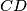$CD$ and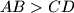$AB > CD$, the line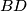$BD$ bisects the angle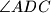$\angle ADC$. The line through$C$ parallel to$AD$ meets the segments$BD$ and$AB$ in$E$ and$F$, respectively. Let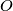$O$ be the circumcenter of the triangle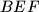$BEF$. Suppose that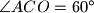$\angle ACO = 60^{\circ}$. Prove the equality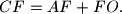Source: Srednjoeuropska matematička olimpijada 2012, pojedinačno natjecanje, problem 3